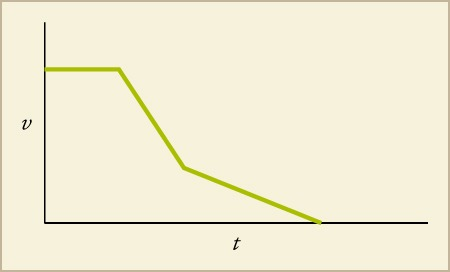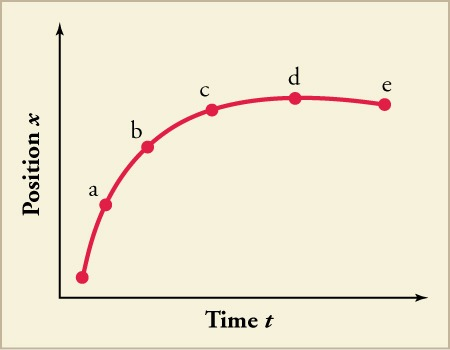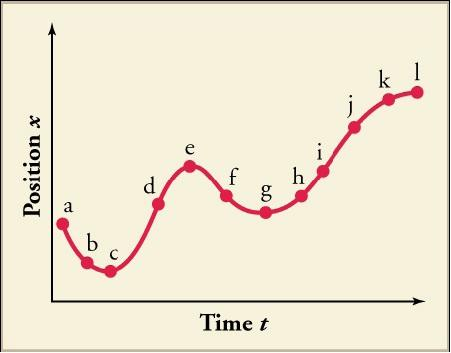Sections
Chapter Review

# Chapter Review

### Concept Items

#### 2.1Relative Motion, Distance, and Displacement

1.
Can one-dimensional motion have zero distance but a nonzero displacement? What about zero displacement but a nonzero distance?
1. One-dimensional motion can have zero distance with a nonzero displacement. Displacement has both magnitude and direction, and it can also have zero displacement with nonzero distance because distance has only magnitude.
2. One-dimensional motion can have zero distance with a nonzero displacement. Displacement has both magnitude and direction, but it cannot have zero displacement with nonzero distance because distance has only magnitude.
3. One-dimensional motion cannot have zero distance with a nonzero displacement. Displacement has both magnitude and direction, but it can have zero displacement with nonzero distance because distance has only magnitude and any motion will be the distance it moves.
4. One-dimensional motion cannot have zero distance with a nonzero displacement. Displacement has both magnitude and direction, and it cannot have zero displacement with nonzero distance because distance has only magnitude.
2.

In which example would you be correct in describing an object in motion while your friend would also be correct in describing that same object as being at rest?

1. You are driving a car toward the east and your friend drives past you in the opposite direction with the same speed. In your frame of reference, you will be in motion. In your friend’s frame of reference, you will be at rest.
2. You are driving a car toward the east and your friend is standing at the bus stop. In your frame of reference, you will be in motion. In your friend’s frame of reference, you will be at rest.
3. You are driving a car toward the east and your friend is standing at the bus stop. In your frame of reference, your friend will be moving toward the west. In your friend’s frame of reference, he will be at rest.
4. You are driving a car toward the east and your friend is standing at the bus stop. In your frame of reference, your friend will be moving toward the east. In your friend’s frame of reference, he will be at rest.
3.
What does your car’s odometer record?
1. displacement
2. distance
3. both distance and displacement
4. the sum of distance and displacement

#### 2.2Speed and Velocity

4.
When discussing an object with a velocity, what physical quantity changes over time?
1. speed
2. distance
3. magnitude of displacement
4. position vector
5.
Which of the following best describes the relationship between instantaneous velocity and instantaneous speed?
1. Both instantaneous speed and instantaneous velocity are the same, even when there is a change in direction.
2. Instantaneous speed and instantaneous velocity cannot be the same even if there is no change in direction of motion.
3. Magnitude of instantaneous velocity is equal to instantaneous speed.
4. Magnitude of instantaneous velocity is always greater than instantaneous speed.

#### 2.3Position vs. Time Graphs

6. Use the graph to describe what the runner’s motion looks like.
Figure 2.25

How are average velocity for only the first four seconds and instantaneous velocity related? What is the runner’s net displacement over the time shown?

1. The net displacement is 12 m and the average velocity is equal to the instantaneous velocity.
2. The net displacement is 12 m and the average velocity is two times the instantaneous velocity.
3. The net displacement is 10 + 12 = 22 m and the average velocity is equal to the instantaneous velocity.
4. The net displacement is 10 + 12 = 22 m and the average velocity is two times the instantaneous velocity.
7.
A position vs. time graph of a frog swimming across a pond has two distinct straight-line sections. The slope of the first section is $1m/s$. The slope of the second section is $0m/s$. If each section lasts $1second$, then what is the frog’s total average velocity?
1. $0m/s$
2. $2m/s$
3. $0.5m/s$
4. $1m/s$

#### 2.4Velocity vs. Time Graphs

8. A graph of velocity vs. time of a ship coming into a harbor is shown below.Describe the acceleration of the ship based on the graph.
1. The ship is moving in the forward direction at a steady rate. Then it accelerates in the forward direction and then decelerates.
2. The ship is moving in the forward direction at a steady rate. Then it turns around and starts decelerating, while traveling in the reverse direction. It then accelerates, but slowly.
3. The ship is moving in the forward direction at a steady rate. Then it decelerates in the forward direction, and then continues to slow down in the forward direction, but with more deceleration.
4. The ship is moving in the forward direction at a steady rate. Then it decelerates in the forward direction, and then continues to slow down in the forward direction, but with less deceleration.

### Critical Thinking Items

#### 2.1Relative Motion, Distance, and Displacement

9.
Boat A and Boat B are traveling at a constant speed in opposite directions when they pass each other. If a person in each boat describes motion based on the boat’s own reference frame, will the description by a person in Boat A of Boat B’s motion be the same as the description by a person in Boat B of Boat A’s motion?
1. Yes, both persons will describe the same motion because motion is independent of the frame of reference.
2. Yes, both persons will describe the same motion because they will perceive the other as moving in the backward direction.
3. No, the motion described by each of them will be different because motion is a relative term.
4. No, the motion described by each of them will be different because the motion perceived by each will be opposite to each other.
10.
Passenger A sits inside a moving train and throws a ball vertically upward. How would the motion of the ball be described by a fellow train passenger B and an observer C who is standing on the platform outside the train?
1. Passenger B sees that the ball has vertical, but no horizontal, motion. Observer C sees the ball has vertical as well as horizontal motion.
2. Passenger B sees the ball has vertical as well as horizontal motion. Observer C sees the ball has the vertical, but no horizontal, motion.
3. Passenger B sees the ball has horizontal but no vertical motion. Observer C sees the ball has vertical as well as horizontal motion.
4. Passenger B sees the ball has vertical as well as horizontal motion. Observer C sees the ball has horizontalbut no vertical motion.

#### 2.2Speed and Velocity

11.
Is it possible to determine a car’s instantaneous velocity from just the speedometer reading?
1. No, it reflects speed but not the direction.
2. No, it reflects the average speed of the car.
3. Yes, it sometimes reflects instantaneous velocity of the car.
4. Yes, it always reflects the instantaneous velocity of the car.
12.

Terri, Aaron, and Jamal all walked along straight paths. Terri walked 3.95 km north in 48 min. Aaron walked 2.65 km west in 31 min. Jamal walked 6.50 km south in 81 min. Which of the following correctly ranks the three boys in order from lowest to highest average speed?

1. Jamal, Terri, Aaron
2. Jamal, Aaron, Terri
3. Terri, Jamal, Aaron
4. Aaron, Terri, Jamal
13.
Rhianna and Logan start at the same point and walk due north. Rhianna walks with an average velocity $vavg,R$. Logan walks three times the distance in twice the time as Rhianna. Which of the following expresses Logan’s average velocity in terms of $vavg,R$?
1. Logan’s average velocity = $1.5vavg,R$.
2. Logan’s average velocity = $23vavg,R$.
3. Logan’s average velocity = $3vavg,R$.
4. Logan’s average velocity = $12vavg,R$.

#### 2.3Position vs. Time Graphs

14. Explain how you can use the graph of position vs. time  to describe the change in velocity over time.Identify the time at which the instantaneous velocity is greatest, the time at which it is zero, and the time at which it is negative.
1. Instantaneous velocity is greatest at $ta$, zero at $td$ and negative at $te$.
2. Instantaneous velocity is greatest at $te$, zero at $td$ and negative at $ta$.
3. Instantaneous velocity is greatest at $td$, zero at $ta$ and negative at $te$.
4. Instantaneous velocity is greatest at $tb$ , zero at $td$ and negative at $te$.

#### 2.4Velocity vs. Time Graphs

15. Identify the time, or times, at which the instantaneous velocity is greatest, and the time, or times, at which it is negative. A sketch of velocity vs. time derived from the figure will aid in arriving at the correct answers.1. The instantaneous velocity is greatest at f, and it is negative at d, h, I, j, and k.
2. The instantaneous velocity is greatest at e, and it is negative at a, b, and f.
3. The instantaneous velocity is greatest at f, and it is negative at d, h, I, j, and k
4. The instantaneous velocity is greatest at d, and it is negative at a, b, and f.

### Problems

#### 2.1Relative Motion, Distance, and Displacement

16.
In a coordinate system in which the direction to the right is positive, what are the distance and displacement of a person who walks $35meters$ to the left, $18meters$ to the right, and then $26meters$ to the left?
1. Distance is $79m$ and displacement is $−43m$.
2. Distance is $−79m$ and displacement is $43m$.
3. Distance is $43m$ and displacement is $−79m$.
4. Distance is $−43m$ and displacement is $79m$.
17.

Billy drops a ball from a height of 1 m. The ball bounces back to a height of 0.8 m, then bounces again to a height of 0.5 m, and bounces once more to a height of 0.2 m. Up is the positive direction. What are the total displacement of the ball and the total distance traveled by the ball?

1. The displacement is equal to -4 m and the distance is equal to 4 m.
2. The displacement is equal to 4 m and the distance is equal to 1 m.
3. The displacement is equal to 4 m and the distance is equal to 1 m.
4. The displacement is equal to -1 m and the distance is equal to 4 m.

#### 2.2Speed and Velocity

18.
You sit in a car that is moving at an average speed of 86.4 km/h. During the 3.3 s that you glance out the window, how far has the car traveled?
1. 7.27 m
2. 79 m
3. 285 km
4. 1026 m

#### 2.3Position vs. Time Graphs

19. Using the graph, what is the average velocity for the whole 10 s?
Figure 2.26
1. The total average velocity is 0 m/s.
2. The total average velocity is 1.2 m/s.
3. The total average velocity is 1.5 m/s.
4. The total average velocity is 3.0 m/s.
20.
A train starts from rest and speeds up for 15 minutes until it reaches a constant velocity of 100 miles/hour. It stays at this speed for half an hour. Then it slows down for another 15 minutes until it is still. Which of the following correctly describes the position vs time graph of the train’s journey?
1. The first 15 minutes is a curve that is concave upward, the middle portion is a straight line with slope 100 miles/hour, and the last portion is a concave downward curve.
2. The first 15 minutes is a curve that is concave downward, the middle portion is a straight line with slope 100 miles/hour, and the last portion is a concave upward curve.
3. The first 15 minutes is a curve that is concave upward, the middle portion is a straight line with slope zero, and the last portion is a concave downward curve.
4. The first 15 minutes is a curve that is concave downward, the middle portion is a straight line with slope zero, and the last portion is a concave upward curve.

#### 2.4Velocity vs. Time Graphs

21.

You are characterizing the motion of an object by measuring the location of the object at discrete moments in time. What is the minimum number of data points you would need to estimate the average acceleration of the object?

1. 1
2. 2
3. 3
4. 4
22. Which option best describes the average acceleration from 40 to 70 s?
Figure 2.27
1. It is negative and smaller in magnitude than the initial acceleration.
2. It is negative and larger in magnitude than the initial acceleration.
3. It is positive and smaller in magnitude than the initial acceleration.
4. It is positive and larger in magnitude than the initial acceleration.
23. The graph shows velocity vs. time.
Figure 2.28

Calculate the net displacement using seven different divisions. Calculate it again using two divisions: 0 → 40 s and 40 → 70 s . Compare. Using both, calculate the average velocity.

1. Displacement and average velocity using seven divisions are 14,312.5 m and 204.5 m/s while with two divisions are 15,500 m and 221.4 m/s respectively.
2. Displacement and average velocity using seven divisions are 15,500 m and 221.4 m/s while with two divisions are 14,312.5 m and 204.5 m/s respectively.
3. Displacement and average velocity using seven divisions are 15,500 m and 204.5 m/s while with two divisions are 14,312.5 m and 221.4 m/s respectively.
4. Displacement and average velocity using seven divisions are 14,312.5 m and 221.4 m/s while with two divisions are 15,500 m and 204.5 m/s respectively.

#### 2.4Velocity vs. Time Graphs

24.

The National Mall in Washington, DC, is a national park containing most of the highly treasured memorials and museums of the United States. However, the National Mall also hosts many events and concerts. The map in Figure 2.32 shows the area for a benefit concert during which the president will speak. The concert stage is near the Lincoln Memorial. The seats and standing room for the crowd will stretch from the stage east to near the Washington Monument, as shown on the map. You are planning the logistics for the concert. Use the map scale to measure any distances needed to make the calculations below.

Figure 2.29

The park has three new long-distance speakers. They would like to use these speakers to broadcast the concert audio to other parts of the National Mall. The speakers can project sound up to 0.35 miles away but they must be connected to one of the power supplies within the concert area. What is the minimum amount of wire needed for each speaker, in miles, in order to project the audio to the following areas? Assume that wire cannot be placed over buildings or any memorials.

Part A. The center of the Jefferson Memorial using power supply 1 (This will involve an elevated wire that can travel over water.)

Part B. The center of the Ellipse using power supply 3 (This wire cannot travel over water.)

Part C. The president’s motorcade will be traveling to the concert from the White House. To avoid concert traffic, the motorcade travels from the White House west down E Street and then turns south on 23rd Street to reach the Lincoln memorial. What minimum speed, in miles per hour to the nearest tenth, would the motorcade have to travel to make the trip in 5 minutes?

Part D. The president could also simply fly from the White House to the Lincoln Memorial using the presidential helicopter, Marine 1. How long would it take Marine 1, traveling slowly at 30 mph, to travel from directly above the White House landing zone (LZ) to directly above the Lincoln Memorial LZ? Disregard liftoff and landing times and report the travel time in minutes to the nearest minute.